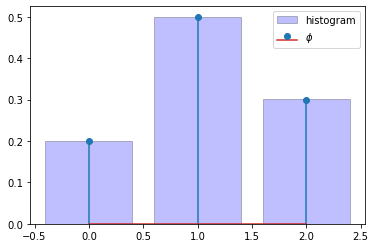# Chapter 2 Code¶

We’ll use Python’s NumPy library in what follows, as well as Matplotlib for plotting.

import numpy as np
import matplotlib.pyplot as plt


## Bisection¶

Here’s an implementation of the bisection algorithm. We will test it on this function:

f = lambda x: np.sin(4 * (x - 1/4)) + x + x**20 - 1


The implementation:

M = 1000
ϵ = 1e-8
α, β = 0, 1

i = 1
a, b = α, β
while i <= M:
c = (a + b) / 2
if abs(f(c)) < ϵ:
print(c)
break
i += 1
if f(a) * f(c) < 0:
b = c
else:
a = c

if i > M:
print("Failed to converge.")

0.408293504267931


## User Defined Functions¶

Here’s the basic implementation of the function $$\tau$$.

def tau(z, S, phi):
"""
Evaluates the function tau(z) given data S, phi, where
S and phi are assumed to be arrays.
"""
a = 0
for i, x in enumerate(S):
b = a + phi[i]
if a < z <= b:
return x
a = b


Here’s a more efficient implementation.

def tau(z, S, phi):
i = np.searchsorted(np.cumsum(phi), z)
return S[i]


And here’s a closure that generates the function $$\tau$$.

def tau_factory(S, phi):
Φ = np.cumsum(phi)

def tau(z):
i = np.searchsorted(Φ, z)
return S[i]

return tau


We generate a function $$\tau$$ that acts on $$z$$ alone by calling the function factory:

phi = 0.2, 0.5, 0.3
S = 0, 1, 2
tau = tau_factory(S, phi)

tau(0.1)  # Should be 0

0


All of these functions work as expected. To illustrate, here $$\tau$$ is used to generate draws from a given distribution $$\phi$$.

size = 100_000

draws = np.empty(size)
for j in range(size):
W = np.random.uniform()
draws[j] = tau(W)

# Compute fraction of draws with each possible value
frequency = [np.mean(draws==j) for j in S]



Let’s check that the empirical frequency approximately coincides with the probabilities in $$\phi$$.

fig, ax = plt.subplots()

ax.bar(S, frequency,
edgecolor='k',
facecolor='b',
alpha=0.25,
label="histogram")

ax.stem(S, phi, label='$\\phi$')

ax.legend()

plt.show()## Object Oriented Programming¶

Here’s a class that implements the function $$\tau$$ as a method, as well as a method to generate draws from $$\phi$$.

class Tau:

def __init__(self, S, phi):
self.S = S
self.Φ = np.cumsum(phi)

def tau(self, z):
i = np.searchsorted(self.Φ, z)
return self.S[i]

def draw(self):
W = np.random.uniform()
return self.tau(W)

tau = Tau(S, phi)

for i in range(5):
print(tau.draw())

0
1
1
1
1